Magnetic Effects of Electric Current - NCERT Questions
Q 1.

Why does a compass needle get deflected when brought near a bar magnet?

SOLUTION:

Compass needle gets deflected due to attractive or repulsive interactions between its magnetic field and the magnetic field of the bar magnet.

Q 2.

Draw magnetic field lines around a bar magnet.

SOLUTION: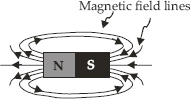Q 3.

List the properties of magnetic lines of force.

SOLUTION:

Some characteristic properties of the magnetic field lines are listed below: (A) Magnetic field lines start at the north pole and end at the south pole. (B) Magnetic field lines do not intersect each other, because there can’t be two directions of the magnetic field at any one point. (C) The degree of closeness of the field lines depends upon the strength of the magnetic field. Stronger the field, closer are the field lines.

Q 4.

Why don’t two magnetic lines of force intersect each other?

SOLUTION:

The direction of magnetic field (B) at any point is obtained by drawing a tangent to the magnetic field line at the point. In case, two magnetic field line intersect each other at the point P as shown in figure, magnetic field at P will have two directions, shown by two arrows, one drawn to each magnetic field line at P, which is not possible.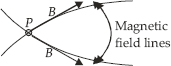Q 5.

Consider a circular loop of wire lying in the plane of the table. Let the current pass through the loop clockwise. Apply the right-hand rule to find out the direction of the magnetic field inside and outside the loop.

SOLUTION:

The magnetic field inside and outside the current-carrying loop is shown in the figure given below.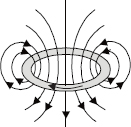Q 6.

The magnetic field in a given region is uniform. Draw a diagram to represent it.

SOLUTION:

The field lines inside the solenoid are in the form of parallel straight lines. This indicates that the magnetic field inside the solenoid is uniform. This is shown in diagram below.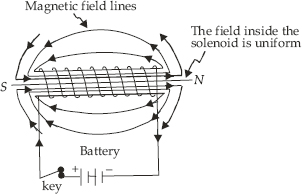Q 7.

The magnetic field inside a long straight solenoid carrying current (A) is zero. (B) decreases as we move towards its end. (C) increases as we move towards its end. (D) is the same at all points.
Choose the correct option.

SOLUTION:

The magnetic field inside a current-carrying solenoid is constant in magnitude and direction, and acts along the axis of the solenoid.

Q 8.

Which of the following property of a proton can change while it moves freely in a magnetic field? (There may be more than one correct answer.)(A) mass       (B) speed (C) velocity       (D) momentum

SOLUTION:

The correct answers are (c) and (d).
Each moving charged particle in a magnetic field experiences a force. The direction of force experienced by a positive charge (i.e., a proton) is given by Fleming’s left hand rule. The force acting on the proton would change both velocity and momentum.

Q 9.

In an activity demonstrating the force acting on a current-carrying conductor placed in a magnetic field as shown in figure how do we think the displacement of rod AB will be affected if (A) current in rod AB is increased? (B) a stronger horse-shoe magnet is used? (C) length of the rod AB is increased?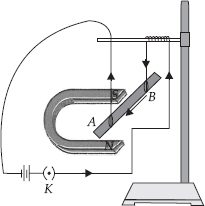SOLUTION:

The displacement of the rod AB (A) will increase when the current in rod AB is increased. (B) will increase when a stronger horse-shoe magnet is used. (C) will increase when length of the rod AB is increased.

Q 10.

A positively-charged particle(alpha-particle)projected towards west is deflected towards north by a magnetic field. The direction of magnetic field is (A) towards south (B) towards east (C) downward (D) upward

SOLUTION:

Apply Fleming’s left-hand rule, we can infer that the direction of magnetic field is upwards.

Q 11.

State Fleming’s left-hand rule.

SOLUTION:

Fleming’s left-hand rule state as follows:
Stretch out the thumb, the forefinger, and the second(middle) finger of the left hand so that these are at right angles to each other. If the forefinger gives the direction of the magnetic field (N to S), the second (middle) finger the direction of current (+ to –), then the thumb gives the direction of the force acting on the conductor.
Since the conductor will move in the direction of the force acting on it hence the thumb gives the direction of motion of the conductor.

Q 12.

What is the principle of an electric motor?

SOLUTION:

An electric motor is based upon the magnetic effect of current. When an electric current is passed through a conductor placed at right angle to a magnetic field, a force perpendicular both to the magnetic field and the conductor acts on it. This makes the conductor move. The direction of motion of the conductor is given by Fleming’s left-hand rule.

Q 13.

What is the role of the split ring in an electric motor?

SOLUTION:

The role of split ring is to change the direction of current flowing through the coil after each half-rotation.

Q 14.

Explain different ways to induce current in a coil.

SOLUTION:

(a) By keeping the magnet in a fixed position and moving the coil towards and away from the magnet.
(b) By pushing or pulling a bar magnet into or away from a coil.

Q 15.

State the principle of an electric generator.

SOLUTION:

Electric generators are based on the principle of electromagnetic induction, that is, when a conductor is moved perpendicular to magnetic field or vice-versa, an induced current is produced.

Q 16.

Name some source of direct current.

SOLUTION:

Q 17.

Which sources produce alternating current?

SOLUTION:

AC generator, Thermal power station, Hydroelectric stations.

Q 18.

Choose the correct option.
A rectangular coil of copper wires is rotated in a magnetic field. The direction of the induced current changes once in each (A) two revolutions (B) one revolution (C) half revolution (D) one fourth revolution

SOLUTION:

Q 19.

Name two safety measures commonly used in electric circuits and appliances.

SOLUTION:

The safety devices that are used in electric circuits and appliances are, (a) Fuse (b) Earthing. MCBs are also used as safety device in electrical circuits.

Q 20.

An electric oven of 2 kW power rating is operated in a domestic electric circuit 220 V that has a current rating of 5 A. What result do you expect? Explain.

SOLUTION:

Rating of the oven = 2 kW
Line voltage = 220 V
Then,
Current drawn by the oven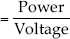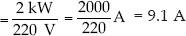= 9.1 A
Since the domestic circuit is rated for 5 A, and the oven draws a current of 9.1 A, the following might result.
(a) The fuse (if there) will blow off.
(b) The wiring may burn out.

Q 21.

What precaution should be taken to avoid the overloading of domestic electric circuits?

SOLUTION:

(a) Do not connect appliances exceeding the total load capacity of the circuit.
(b) Provide fuses/MCBs of proper rating.

Q 22.

Which of the following correctly describes the magnetic field near a long straight wire? (A) The field consists of straight line perpendicular to the wire. (B) The field consists of straight lines parallel to the wire. (C) The field consists of radial lines originating from the wire. (D) The field consists of concentric circles centred on the wire.

SOLUTION:

The correct option is (d).

Q 23.

The phenomena of electromagnetic induction is (A) the process of charging a body (B) the process of generating magnetic field due to a current passing through a coil (C) producing induced current in a coil due to relative motion between a magnet and the coil (D) the process of rotating a coil of an electric motor

SOLUTION:

The correct option is (c).

Q 24.

The device used for producing electric current is called a: (A) generator (B) galvanometer (C) ammeter (D) motor

SOLUTION:

The correct option is (a).

Q 25.

The essential difference between AC generator and a DC generator is that (A) AC generator has an electromagnet while a DC generator has permanent magnet, (B) DC generator will generate a higher voltage, (C) AC generator will generate a higher voltage, (D) AC generator has slip rings while the DC generator has a commutator.

SOLUTION:

The correct option is (d).

Q 26.

At the time of short-circuit, the current in the circuit (A) reduces substantially (B) does not change (C) increases heavily (D) varies continuously.

SOLUTION:

Q 27.

State whether the following statements are true or false. (A) An electric motor converts mechanical energy into electrical energy. (B) An electric generator works on the principle of electromagnetic induction. (C) The field at the centre of a long circular coil carrying current will be parallel straight lines. (D) A wire with a green insulation is usually the live wire.

SOLUTION:

(a) False: An electric motor converts electric energy into mechanical energy.
(b) True
(c) True
(d) False: A wire with a green insulation is usually the earth wire.

Q 28.

List three sources of magnetic fields.

SOLUTION:

(i) Natural and artificial magnets (ii) Electromagnets (iii) A conductor, a coil and a solenoid carrying current (iv) Earth.

Q 29.

How does a solenoid behave like a magnet? Can you determine the north and south poles of a current carrying solenoid with the help of a bar magnet? Explain.

SOLUTION:

A current carrying solenoid behaves like a bar magnet and the polarities of its ends depend upon the direction of current flowing through it. In order to determine the polarities of its ends, place it in a brass hook and suspend it with a long thread so that it moves freely. Bring north pole of a bar magnet near one of its ends. In case the solenoid moves towards the bar magnet, that end of the solenoid is a south pole and in case the solenoid moves away from the magnet, that end of the solenoid is its north pole. The polarity of the other end of the solenoid can similarly be determined.

Q 30.

When is the force experienced by current-carrying conductor placed in a magnetic field is largest?

SOLUTION:

The force experienced by a current-carrying conductor placed in a magnetic field is the largest when the direction of the current is at right angles to the direction of the magnetic field.

Q 31.

Imagine that you are sitting in a chamber with your back to one wall. An electron beam, moving horizontally from back wall towards the front wall, is deflected by a strong magnetic field to your right side. What is the direction of the magnetic field?

SOLUTION:

The direction of current I is opposite to the direction electron beam as shown in figure. Since the beam is deflected to the right side, the force, F acting on the beam is as shown. Applying Fleming’s left hand rule, it is found that magnetic field, B is acting vertically downwards (i.e., perpendicular to the plane of the paper and directed inwards) as shown by ⊗.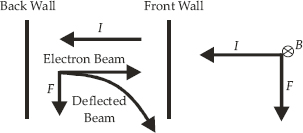Q 32.

Draw a labelled diagram of an electric motor. Explain its principle and working. What is the function of a split-ring in an electric motor?

SOLUTION:

An electric motor is a device for converting electric energy into mechanical energy. Thus, an electric motor is the reverse of an electric generator.
There are two types of electric motors : (i) AC motor and (ii) DC motor. We shall here be describing DC motor. The principle of a DC motor is very much different from that of an AC motor. It is important to remember that all the electric appliances like fan, air-conditioner, coolers, washing machines, mixers and blenders work on AC (house-hold power supply) and as such have AC motors installed in them.
Principle : When a coil carrying current is placed in a magnetic field, it experiences a torque. As a result of this torque, the coil begins to rotate.
Construction : It consists of the following five parts:
1. Armature : The armature abcd consists of a large number of turns of insulated copper wire wound over a soft iron core.
2. Field Magnet : The magnetic field B is supplied by a permanent magnet NS.
3. Split-ring or Commutator : These are two halve of the same metallic ring. The ends of the armature coil are connected to these halves which also rotate with the armature.
4. Brushes or Sliding Contacts : These are two flexible metal plates or carbon rods B1 and B2 which are so fixed that they constantly touch the revolving rings.
5. Battery : The battery consists of a few cells and is connected across the brushes. The brushes pass the current to the rings from where it is carried to the armature.
Working : The working of a DC motor will be clear from the following discussion.
(a) Let us suppose that the battery sends current to the armature in the direction shown in figure. Applying Fleming’s Left Hand Rule (Motor Rule), we find that arm ad experiences a force which is acting outwards and perpendicular to it and arm bc experiences a force which is acting inwards and perpendicular to it. These two forces form a couple whose moment (i.e., torque) makes the armature rotate in the clockwise direction.
(b) After the armature has completed half a revolution (i.e., has turned through 180°), the direction of current in the arms ad and bc is reversed. Now arm bc experiences an outward force and arm ad experiences an inward force, as shown in figure. The armature thus continues to rotate about its axis in the same, i.e., clockwise direction.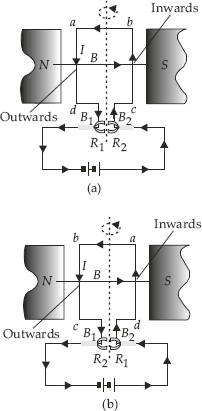Split-ring in an electric motor takes the current from the battery and passes it on to the coil through the brushes after reversing its direction after every half revolution. The reversal of current in the coil reverses the direction of forces acting on the sides of the loop.

Q 33.

Name some devices in which electric motors are used.

SOLUTION:

Electric fans, water-pumps, coolers, refrigerators, mixers, blenders, washing machines etc.

Q 34.

A coil of insulated copper wire is connected to a galvanometer. What would happen if a bar magnet is
(i) pushed into the coil?
(ii) withdrawn from inside the coil?
(iii) held stationary inside the coil?

SOLUTION:

(i) The magnetic field lines (flux) linked with the coil changes (i.e., increases). As a result of this, an induced current flows in the coil and the galvanometer shows a momentary deflection(say towards right) i.e., the needle of the galvanometer moves momentarily in one direction.
(ii) The magnetic field lines (flux) linked with the coil changes (i.e., decreases). As a result of this, an induced current flows in the coil but in a direction opposite to that in case (i).
(iii) When the magnet is held stationary in the coil, there will be a magnetic flux in the coil but it will remain constant. Since the magnetic flux does not change, there is no induced current in the coil and the galvanometer shows no deflection.

Q 35.

Two circular coils A and B are placed close to each other. If the current in the coil A is changed, will some current be induced in the coil B? Given reason.

SOLUTION:

By changing current in the coil A, a current will be induced in the coil B which is placed close to A. This is due to the reason that magnetic field lines linked with A also get linked with B due to its being close to A. When the current in A changes, magnetic field lines linked with A change. Obviously, magnetic field lines linked with B also change. As a result of this, current is induced in the coil B. (This phenomenon is called mutual induction).

Q 36.

State the rule to determine the direction of a
(i) magnetic field produced around a straight conductor carrying current.
(ii) force experienced by a current-carrying straight conductor placed in a magnetic field which is perpendicular to it, and
(iii) current induced in a coil due to its rotation in a magnetic field.

SOLUTION:

(i) Right-Hand Thumb Rule : Imagine the straight conductor in your right hand such that the thumb points in the direction of current. The direction of curling of fingers of the right hand gives the direction of magnetic field lines.
(ii) Fleming’s Left-Hand Rule (or Motor rule): Stretch the thumb, the first finger and the central finger of the left hand so that they are mutually perpendicular to each other. If the first (fore)finger points in the direction of the magnetic field, the central finger points in the direction of current, then the thumb points in the direction of motion of the conductor (i.e., direction of force on the conductor).
(iii) Fleming’s Right-Hand Rule (or Dynamo rule) : Stretch the thumb, the first finger and the central finger of the right hand so that they are mutually perpendicular to each other. If the first(fore)finger points in the direction of magnetic field, the thumb points in the direction of motion of the conductor, then the central finger points in the direction of induced current.

Q 37.

Explain the underlying principle and working of an electric generator by drawing a labelled diagram. What is the function of brushes?

SOLUTION:

An AC generator converts mechanical energy into electric energy.
Principal : Whenever in a closed circuit (i.e., a coil), the magnetic field lines changes, an induced current is produced.
Construction : It consists of the following four parts.
1. Armature : Armature (ABCD), also called the coil, consists of a large number of turns of insulated copper wire would over a soft iron core. It revolves around an axle between the two poles of a strong magnet.
2. Field Magnet : The magnetic field (B) is supplied by a permanent magnet in a small dynamo (also called a magneto) and by an electromagnet in case of a big commercial dynamo (usually called a generator). The poles of the magnet are shown as N-S in figure (a).
3. Slip Rings : R1 and R2 are two hollow metal rings held at different heights. The end D of the armature coil is connected to ring R1. The end C of the coil is passed through R1 without touching it and is connected to R2. These rings rotate with the rotation of the armature.
4. Brushes or Sliding Contact : B1 and B2 are flexible metal plates or carbon rods. These are called brushes or sliding contacts. B1 is in constant touch with R1 and B2 is in constant touch with R2. It is with the help of these brushes that the induced current is passed on from the armature and the rings to the external circuit containing a resistance, R and a galvanometer, G. Brushes are stationary i.e. these do not rotate with the rotation of the armature.
Working : The working of an AC generator is clear from figure (a) and (b). As the armature is rotated about an axis (shown dotted), the magnetic flux linked with the armature changes. Therefore, an induced current is produced in the armature.
(a) Let us suppose that the armature abcd is rotating anticlockwise so that the arm AD moves inwards and BC moves outwards. Applying Fleming’s right-hand rule, we find that the induced current in the armature and in the circuit is as shown in Fig. (a) due to which G shows deflection towards the right.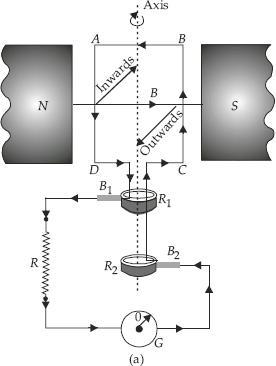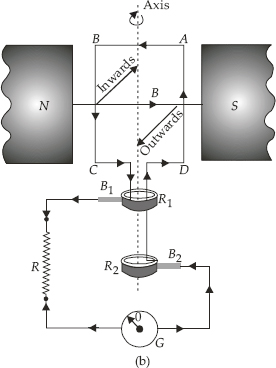(b) After the armature has turned through 180°, it occupies the position shown in Fig. (b). With the armature rotating in the same direction (i.e., anticlockwise), bc moves inwards and ad moves outwards. Thus, again applying Fleming’s right-hand rule, we find the induced current in the external circuit (R and G) flows in the opposite direction due to which the direction of deflection in the galvanometer is towards left.
Thus, we see that the direction of induced current changes in external circuit after every half revolution of the armature, i.e., after the armature has turned through an angle of 180° from its initial position. Hence, the induced current is alternating in nature.

Q 38.

When does an electric short-circuit occur?

SOLUTION:

Electric short-circuit occurs when the live wire and the neutral wire come in direct contact. This occurs when
(i) the insulation of wires is damaged or
(ii) there is a fault in the electric appliance.

Q 39.

What is the function of an earth wire? Why is it necessary to earth metallic appliances?

SOLUTION:

Many electric appliances of daily use like electric press, heater, toaster, refrigerator, table fan etc. have a metallic body. If the insulation of any of these appliances melts and makes contact with the metallic casing, the person touching it is likely to receive a severe electric shock. This is due to the reason that the metallic casing will be at the same potential as the applied one. Obviously, the electric current will flow through the body of the person who touches the appliance. To avoid such serious accidents, the metal casing of the electric appliance is earthed. Since the earth does not offer any resistance, the current flows to the earth through the earth wire instead of flowing through the body of the person.